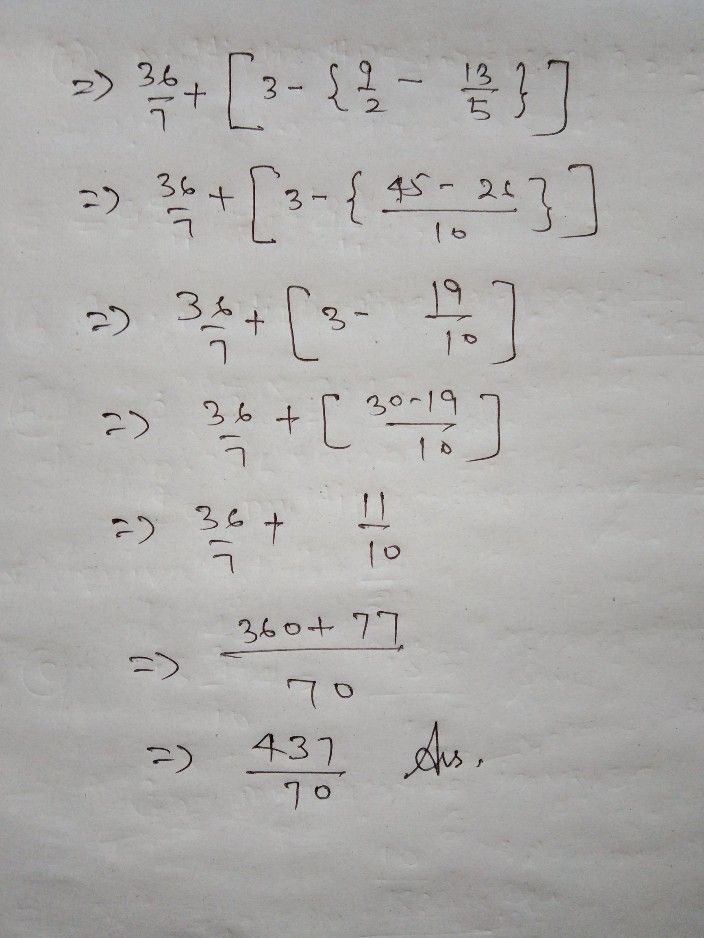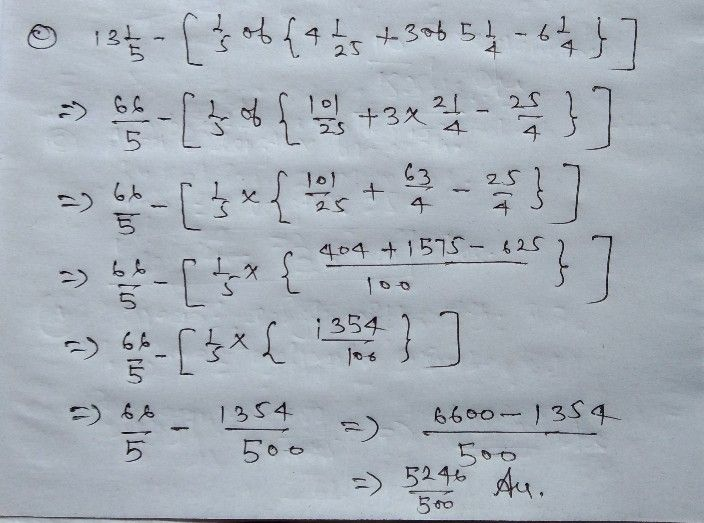Symbol
ProblemSimplify the following expressions: a) $5\dfrac {1} {7}+\left(3-\left(4\dfrac {1} {2}-\left(\dfrac {1} {4}$ of $1\dfrac {1} {3}+2\dfrac {1} {5}+1\right)\right)$ c) $13\dfrac {1} {5}-\left(\dfrac {1} {5}$ of $C$ $\left(4\dfrac {1} {25}+3$ of $f5\dfrac {1} {4}-6\dfrac {1} {4}\right)\right)$ Think
Calculus
SolutionQanda teacher - DharmendraStudent
Thank you sirQanda teacher - Dharmendra
welcome
if u r satisfied with my solution so please rate me 5 star....